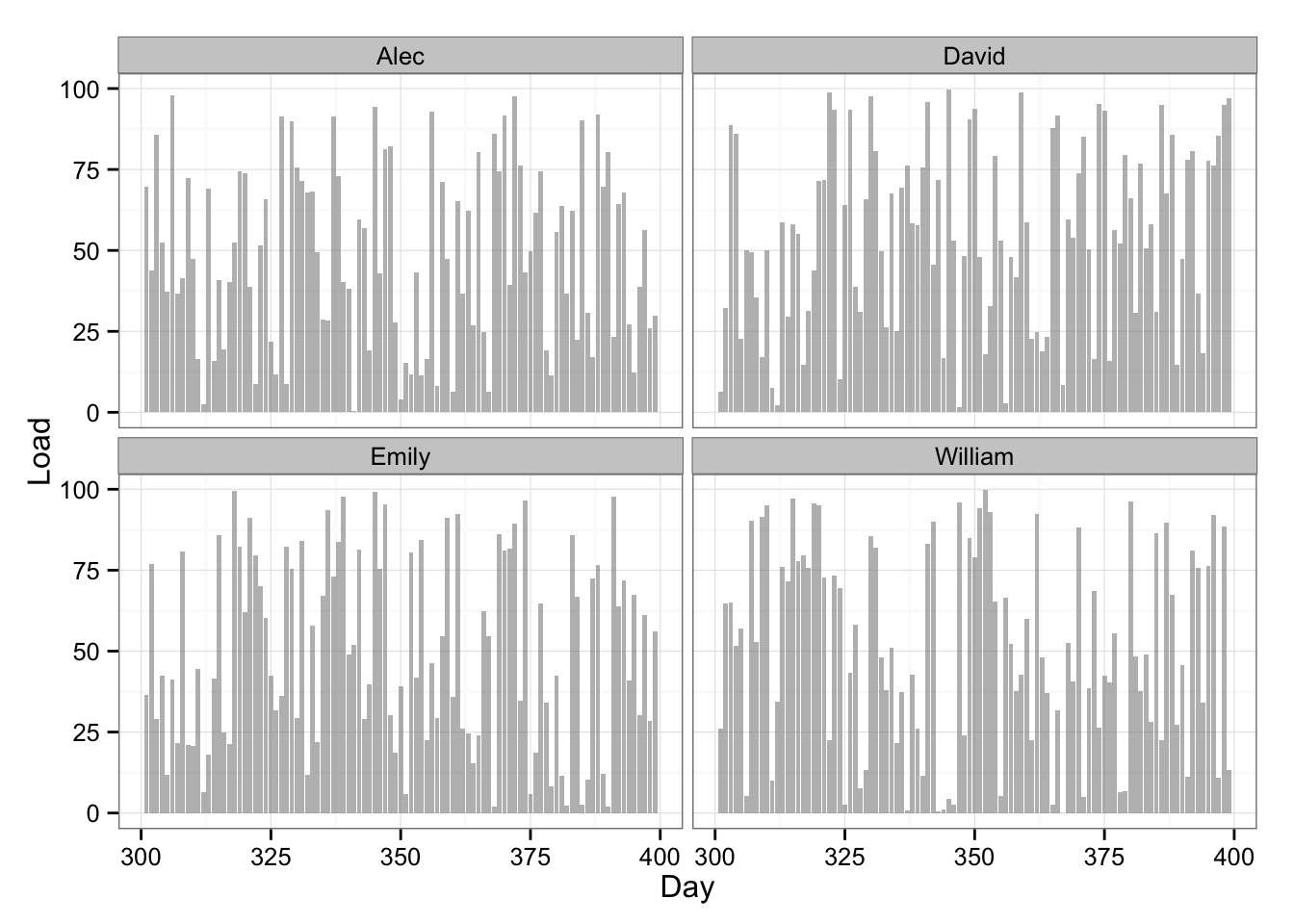# R Playbook: Injury Prediction using Random Forest

This is the problem I have been wrestling with for some time and I tried to solve it using Bannister model. I even asked for help on Twitter and couple of data scientists suggested taking a look into “functional data analysis” or creating summary variables and performing regression/classification (which I did here). Here is my Twitter post:

The problem I am looking for analyzing are predicting injury data for training load data. The basic structure involves multivariate time series (e.g. for every day and every player we track multiple training load metrics: training duration, distance covered, high intensity running and so forth - can reach >200 metrics) and TRUE/FALSE injury target variable (for occurrence) or days lost (for well - lost days). There are multiple ways to express “injury” occurrence/loss time, which I won’t go here.

What we are trying to do is to create injury risk/probability model for a single athlete (and/or team) from this multivariate time series data. I created a video I did using Banister model in analyzing very simple data set (single load metric), but failed to “constrain” injury probability with logit(stic) model. Here is the video: https://www.youtube.com/watch?v=7JqLafOR67Y

The problems are the following:

1. I am trying to find a particular method in analyzing this data set - something that predicts from multivariate time series (just throwing things in regression will not help, since the order of data is important). Besides, if we change Injury target variable to performance (which is continuous), how can we predict one time series fro other multiple time series? Banister models works in this realm, but I am looking for other options (there is PerPot model by Perl).

1. You need injuries to predict injuries. And some players don’t have them in the data set (which is good). So we need to use “hierarchical” model and try to make inferences on a individual from the group data (“Bayesian hierarchical” ?) e.g. apriori.

Any help with this is highly appreciate.

As I mentioned in the above post, I beleive that some type of Bayesian Hierarchical time-series analysis should be applied, but have no clue if such thing exists and how to apply it. So I tried to play with data summaries and performing more “traditional” approach (e.g. not time-series analysis).

For the sake of this playbook I will simulate some data for 60 athletes and 1000 days duration with couple of injuries. Since this is a lot of data, I will display only 100 data points for couple of athletes, but perform analysis on everyone.

Ok, let’s generate some data and display it in the table

``````# Load libraries
library(randomNames)
library(dplyr)
library(ggplot2)
library(reshape2)
library(zoo)
library(rpart)
library(DMwR)
library(randomForest)

# Generate data
set.seed(100)
numberOfPlayers <- 60
numberOfDays <- 1000

playersData <- data.frame(Name = rep(randomNames(numberOfPlayers, which.names = "first"), numberOfDays),
Day = rep(1:numberOfDays, each = numberOfPlayers),
Load = runif(numberOfPlayers * numberOfDays, min = 0, max = 100))

playersDataPrint <- playersData %>%
filter(Day > 300,
Day < 400,
Name == "Alec" | Name == "David" | Name == "Emily" | Name == "William" )``````

Here is the same data plotted:

``````# Plot the data
gg <- ggplot(playersDataPrint, aes(x = Day, y = Load)) +
geom_bar(stat = "identity", fill = "#8C8C8C", alpha = 0.6) +
facet_wrap(~Name) +
theme_bw()
gg``````What we need to do now is to create some summary metrics. I decided to do 7,14,21,28,35,42 rolling averages/sum/sd for each athlete. I started using dplyr package which is great for data wrangling

``````# Engineer the metrics
for (summaryFunction in c("mean", "sd", "sum")) {
for ( i in seq(7, 42, by = 7)) {
tempColumn <- playersData %>%
group_by(Name) %>%
width = i,
FUN = summaryFunction,
align = "right",
fill = NA,
na.rm = T))
colnames(tempColumn) <- paste("Rolling", summaryFunction, as.character(i), sep = ".")
playersData <- bind_cols(playersData, tempColumn)
}
}``````

Since rolling data summaries will leave NAs for rows which rolling window doesn’t take into consideration, I decided to trim our data to leave out NAs.

``````# Cut out first 100 days to avoid NAs in RandomForest later
playersData <- playersData %>%
filter(Day > 100) %>%
mutate(Day = Day - 100)

# Create shorter data frame for visualizing
playersDataPrint <- playersData %>%
filter(Day > 300,
Day < 400,
Name == "Alec" | Name == "David" | Name == "Emily" | Name == "William" )``````

Here is the table of the data and plot with 7 and 42 rolling average:

``````# Plot the data with two rolling averages
gg <- ggplot(playersDataPrint, aes(x = Day, y = Load)) +
geom_bar(stat = "identity", fill = "#8C8C8C", alpha = 0.6) +
geom_line(aes(y = Rolling.mean.7, x = Day), color = "#5DA5FF", size = 1) +
geom_line(aes(y = Rolling.mean.42, x = Day), color = "#60BD68", size = 1) +
facet_wrap(~Name) +
theme_bw()
gg``````